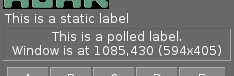<-- Back to AG_Intro.3

# SYNOPSIS

 ```#include #include #include ```

# DESCRIPTIONThe formatting engine used by AG_Printf(3) and AG_PrintfP(3) allow the registration of format string extensions. Once the Agar-Math library is initialized, the following format specifiers become available:
 %[R] Real-number (of M_Real(3) type). %[T] A time value (of M_Time(3) type). %[C] A complex number (of M_Complex(3) type). %[V] A general vector in Rn (of M_Vector(3) type). %[V2] A vector in R2 (of M_Vector2 type). %[V3] A vector in R3 (of M_Vector3 type). %[V4] A vector in R4 (of M_Vector4 type). %[M] A general matrix (of M_Matrix(3) type). %[M44] A 4x4 matrix (of M_Matrix44 type).